# A sum

A sum of money is shared between Peter, John and Henry in the ratio 2:3:5.
a) express Henry's share as fraction of John's share.
b) what fraction of the whole sum of money is John's share?

a =  1.6667
J =  0.3

### Step-by-step explanation: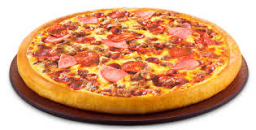Did you find an error or inaccuracy? Feel free to write us. Thank you!Tips to related online calculators
Need help to calculate sum, simplify or multiply fractions? Try our fraction calculator.

## Related math problems and questions:

• Division by zero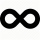Fraction 5 by 2. if 3 is added to numerator and 2 is subtracted from the denominator then the new fraction is:
• Ratio three numbers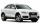Three numbers SUV are in the ratio 1:2:3. Their sum is 24. Find this numbers and write their add and sum.
• Ratio6 numbers are in the ratio 1:5:1:5:5:5. Their sum is 242. What are the numbers?
• Cake 71/3 of a cake shared with 4 people. What share of the whole cake has each people?
• A pizza 2A pizza is cut into slices that are each 1/6 of the whole. John is going to eat 1/2 of the whole pizza. How many slices will John eat?
• ErikThree friends Peter, Erik and Milan shared 420 euros in a ratio of 1: 2: 3. How many euros did Erik get?
• Divide moneyDivide 1200 USD at a ratio of 1:2:3:4:5:6:9:10
• Euros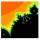Michal, Peter, John and Lenka got together 2,400 euros. They share an amount in ratio 2:6:4:3. How many got each of them?
• Between two mixed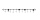What is the rational number between 2 1/4 and 2 4/5?
• Sum of fractionsWhat is the sum of 2/3+3/5?
• The sum 12The sum of 3 mixed numbers is 20 13/15. two of the numbers are 6 1/3 and 7 5/6. what is the third number?
• Moneys in triple ratioMilan, John and Lili have a total 344 euros. Their amounts are in the ratio 1:2:5. Determine how much each of them has?
• JerryJerry has 3/4 of a pizza. He needs to share it with 6 friends. What fraction of the pizza will each friend get? Only write the fraction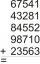Fill letters instead of digits so the indicated sum (equal letters represent equal digits). What number is hidden under the letter J? A A H A H O A H O J -------------------------- 4 3 2 1(a) Convert the following mixed numbers to improper fractions. i. 3 5/8 ii. 7 7/6 (b) Convert the following improper fraction to a mixed number. i. 13/4 ii. 78/5 (c) Simplify these fractions to their lowest terms. i. 36/42 ii. 27/45 2. evaluate the followWhen 5 is subtracted from both my numerator and denominator I become 3/4. What fraction i am?A land area was divided among the three heirs in the ratio 5:2:4. If the largest share was 20 hectares of land, what is the total area of land? Please show your solution and what kind of proportion is this please